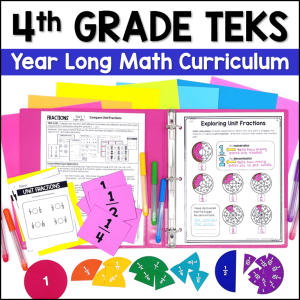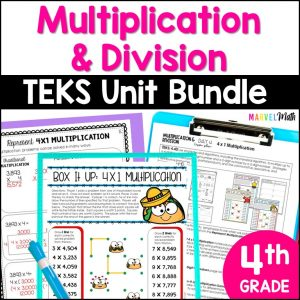Save 10% on your first order with code EXTRA10

# 4th Grade Multiplication & Division Worksheets

\$3.00187.25\$30.99
SKU: 4575270 Category:

## Description

This resource includes 28 student printables that cover Multiplication and Division of Whole Numbers. Fun & engaging student pages cover the Area Model, Distributive Property, Partial Products, as well as Traditional Multiplication and Division. Several pages integrate input-output tables and measurement conversions to give students meaningful practice and make essential connections between the standards.

The TEKS covered by each worksheet are listed in the table of contents as well as on each page to make planning easier!

?Printables cover these TEKS?

4.4B Determine products of a number and 10 or 100 using properties of operations and place value understandings

4.4C Represent the product of 2 two-digit numbers using arrays, area models, or equations, including perfect squares through 15 by 15

4.4D Use strategies and algorithms, including the standard algorithm, to multiply up to a four-digit number by a one-digit number and to multiply a two-digit number by a two-digit number. Strategies may include mental math, partial products, and the commutative, associative, and distributive properties

4.4E Represent the quotient of up to a four-digit whole number divided by a one-digit whole number using arrays, area models, or equations

4.4F Use strategies and algorithms, including the standard algorithm, to divide up to a four-digit dividend by a one-digit divisor

4.4H Solve with fluency one- and two-step problems involving multiplication and division, including interpreting remainders